## 正文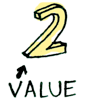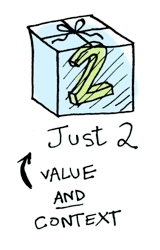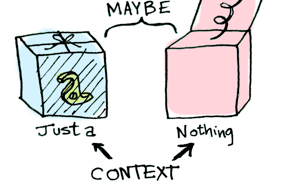``````data Maybe a = Nothing | Just a
``````

## Functors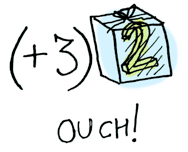``````> fmap (+3) (Just 2)
Just 5
``````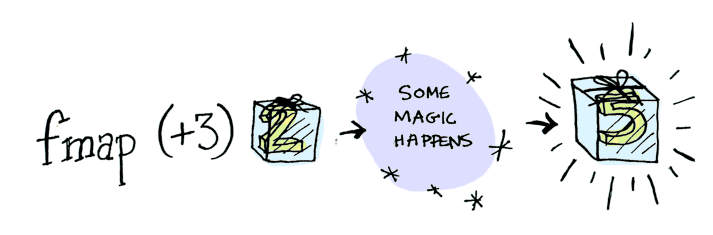## Functor 究竟是啥

`Functor` 是一个 typeclass，这是它的定义：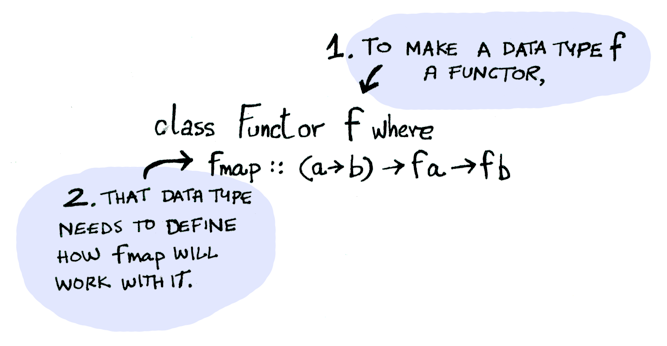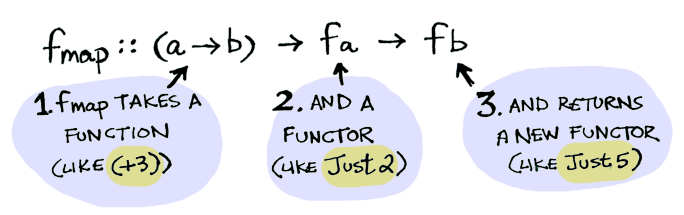``````> fmap (+3) (Just 2)
Just 5
``````

``````instance Functor Maybe where
fmap func (Just val) = Just (func val)
fmap func Nothing = Nothing
``````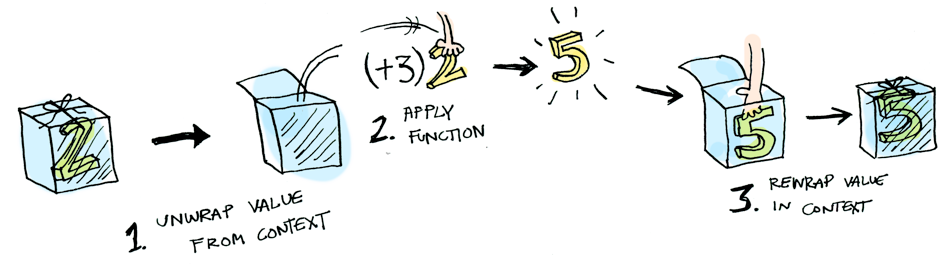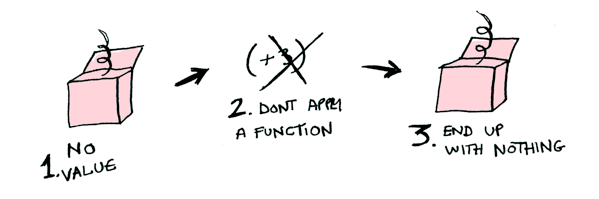``````> fmap (+3) Nothing
Nothing
````````````post = Post.find_by_id(1)
if post
return post.title
else
return nil
end
``````

``````fmap (getPostTitle) (findPost 1)
``````

``````getPostTitle <\$> (findPost 1)
``````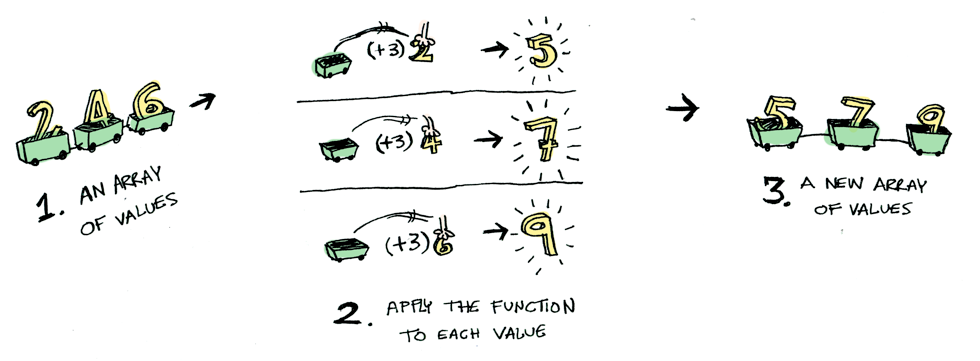``````instance Functor [] where
fmap = map
``````

``````fmap (+3) (+1)
``````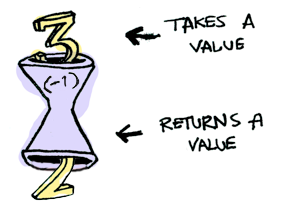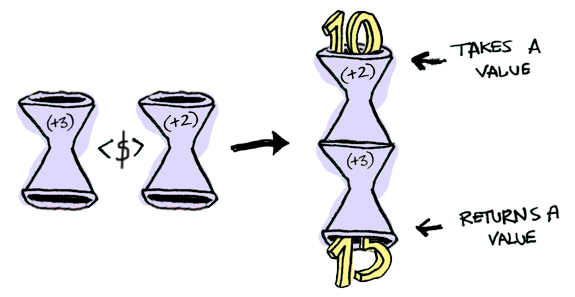``````> import Control.Applicative
> let foo = fmap (+3) (+2)
> foo 10
15
``````

``````instance Functor ((->) r) where
fmap f g = f . g
``````

## Applicatives

`Applicative``Functor` 提高了一个层次。与 `Functor` 类似，我们的值，被放在一个上下文里：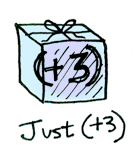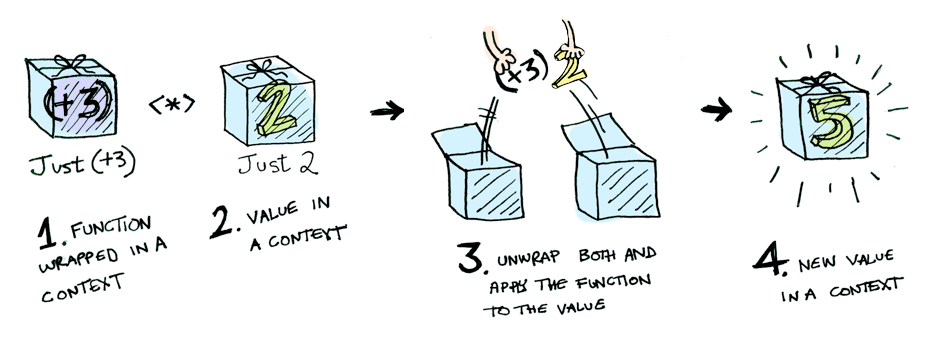``````> [(*2), (+3)] <*> [1, 2, 3]
[2, 4, 6, 4, 5, 6]
``````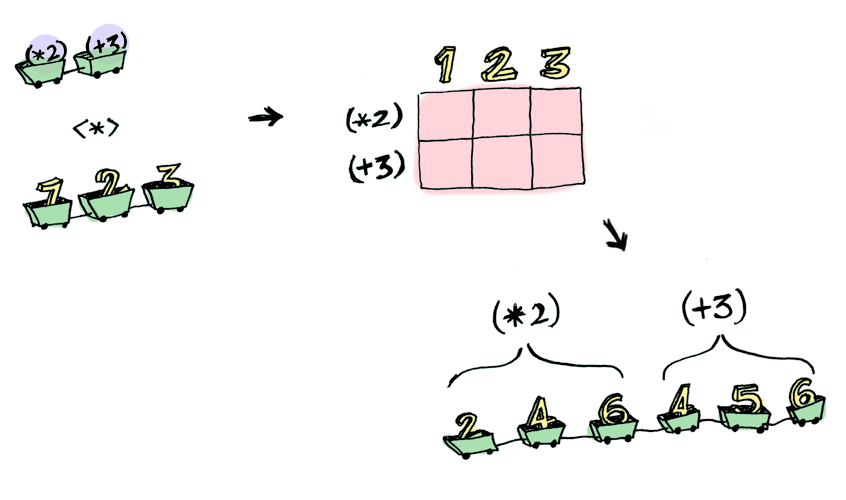``````> (+) <\$> (Just 5)
Just (+5)
> Just (+5) <\$> (Just 4)
ERROR ??? WHAT DOES THIS EVEN MEAN WHY IS THE FUNCTION WRAPPED IN A JUST
``````

``````> (+) <\$> (Just 5)
Just (+5)
> Just (+5) <*> (Just 3)
Just 8
``````

`Applicative` 击败了 `Functor`。“大人可以使用任意数量参数的函数，”它说。“装备了 `<\$>``<*>`，我可以接受任何函数，它们接受任意数量上下文里的值。然后，我将值传入，并得到一个传出的值！哈哈哈哈！”

``````> (*) <\$> Just 5 <*> Just 3
Just 15
``````

``````> liftA2 (*) (Just 5) (Just 3)
Just 15
``````

1. 读一个计算机科学的 PhD。
2. 抛之脑后，不要想它。因为在本节你不需要它！

`Functor` 为上下文里的值，应用一个函数：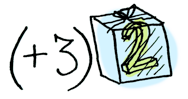`Applicative` 为上下文里的值，应用一个上下文里的函数：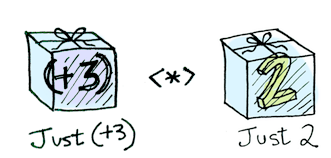`Monad` 为上下文里的值，应用一个接受普通值、返回上下文值的函数。它有一个 `>>=`（读作 “bind”）函数做到这点。 让我们看一个例子。我们熟悉的 `Maybe`，它也是个 Monad：``````half x = if even x
then Just (x `div` 2)
else Nothing
``````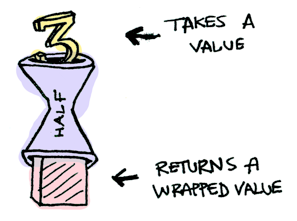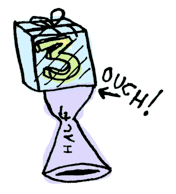``````> Just 3 >>= half
Nothing
> Just 4 >>= half
Just 2
> Nothing >>= half
Nothing
``````

``````class Monad m where
(>>=) :: m a -> (a -> m b) -> m b
``````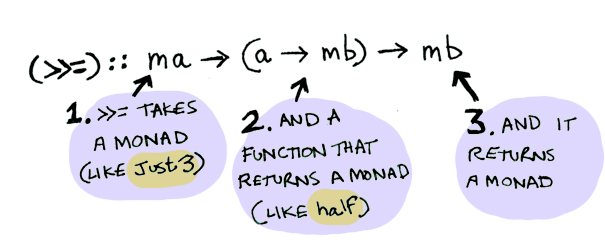``````instance Monad Maybe where
Nothing >>= func = Nothing
Just val >>= func  = func val
``````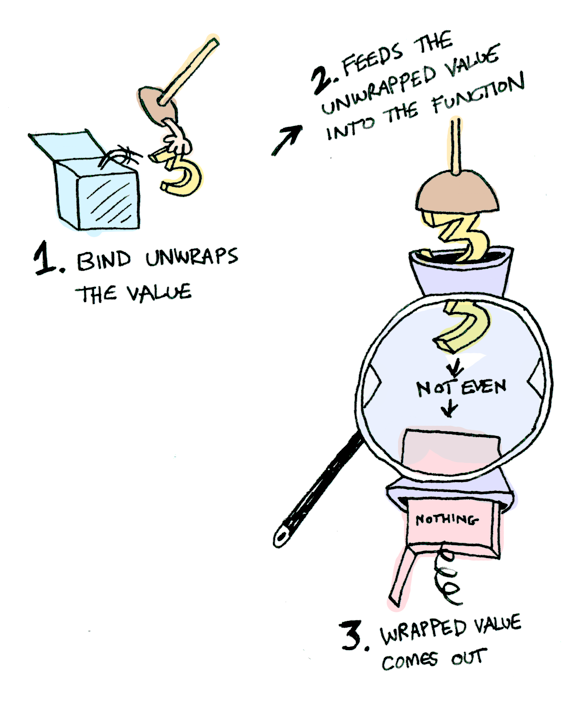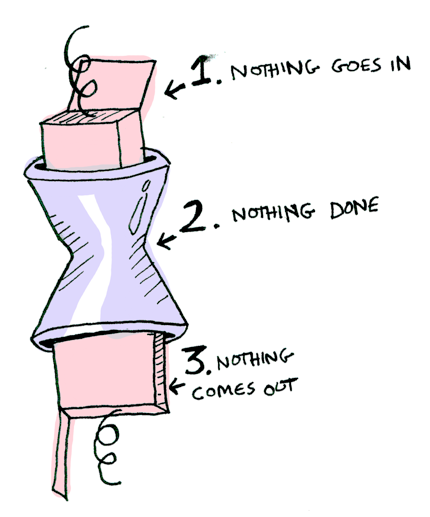``````> Just 20 >>= half >>= half >>= half
Nothing
``````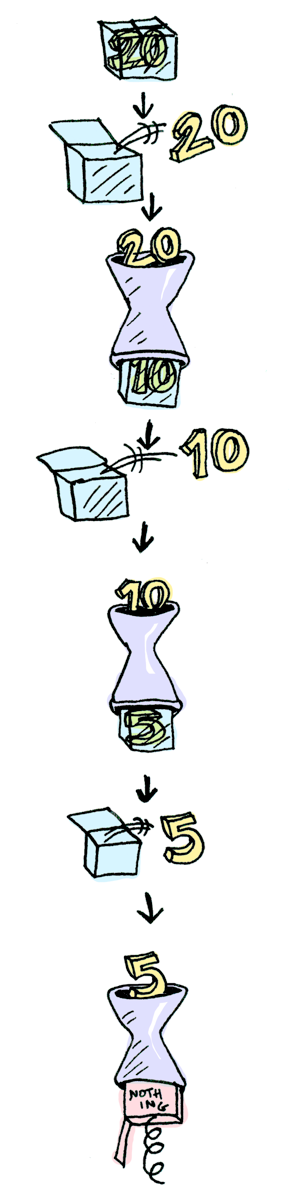Cool！目前为止，我们知道 `Maybe` 是一个 Functor、一个 Applicative，还是个 Monad。 现在，让我们转向另一个例子：`IO` monad：``````getLine :: IO String
``````

`readFile` 接收一个 `string` 类型的文件名，并返回文件的内容：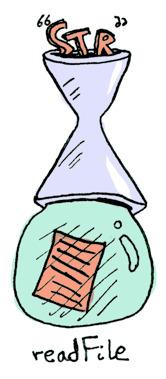``````readFile :: FilePath -> IO String
``````

`putStrLn` 接收一个 `string`，并将其打印：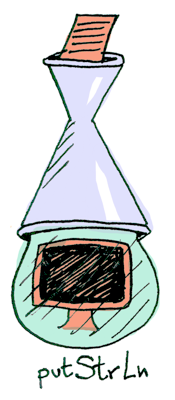``````putStrLn :: String -> IO ()
``````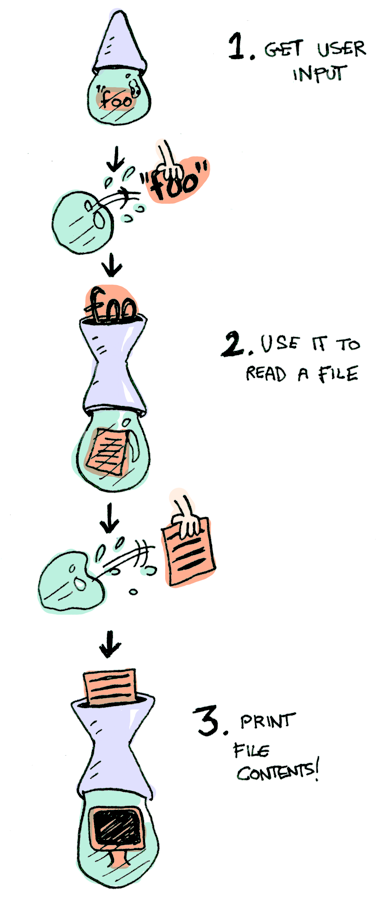``````getLine >>= readFile >>= putStrLn
``````

``````foo = do
filename <- getLine
putStrLn contents
``````

## 结论

1. functor 是实现 `Functor` typeclass 的数据类型
2. applicative 是实现 `Applicative` typeclass 的数据类型
3. monad 是实现 `Monad` typeclass 的数据类型
4. `Maybe` 实现了所有它们三个，因此是一个 functor、applicative，和 monad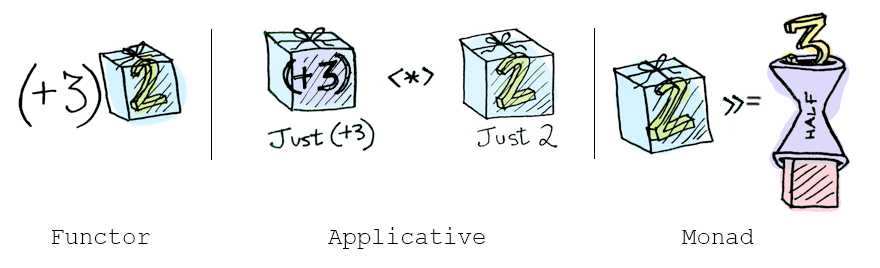• functors：使用 `fmap``<\$>`，为上下文里的值，应用一个函数
• applicatives：使用 `<*>``liftA`，为上下文里的值，应用一个上下文里的函数
• monads：使用 `>>=``liftM`，为上下文里的值，应用一个接受普通值、返回上下文值的函数